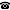# 2012 seminar talk: Analyzing the Complexity of Wild Knot Equivalence

Talk held by Vadim Kulikov (KGRC) at the KGRC seminar on 2012-10-04.

### Abstract

A wild knot is an embedding of the unit circle into the three dimensional Euclidean space $\mathbb{R}^3$, or more conventionally its one-point compactification $S^3$. Two knots $f$ and $g$ are defined to be equivalent, if there exists an orientation preserving homoemorphism $H$ of $S^3$ onto itself that takes one knot to another: either $H\circ f = g$ or just $range(H\circ f)=range(g)$. We show that the isomorphism relation on all countable structures (in a finite vocabulary) is continuously reducible to this equivalence relation which provides a lower bound for the complexity of wild knot equivalence.

Kurt Gödel Research Center for Mathematical Logic. Währinger Straße 25, 1090 Wien, Austria.+43-1-4277-50501. Last updated: 2010-12-16, 04:37.